﻿ Application of Eigenvalues and Eigenvectors and Diagonalization to Environmental Science

### Application of Eigenvalues and Eigenvectors and Diagonalization to Environmental Science

Tanvir Prince, Nieves AnguloOPEN ACCESSPEER-REVIEWED

## Application of Eigenvalues and Eigenvectors and Diagonalization to Environmental Science

Tanvir Prince1,, Nieves Angulo1

1Department of Mathematics, Hostos Community College, City University of New York, 500 Grand Concourse, Bronx, New York

### Abstract

Eigenvalues and Eigenvectors are usually taught toward the middle of the semester and this modulo can be implemented right after the topics of diagonalization. Comparing to the other modulo, students will see applications of some advance topics. This also shows one quick application of eigenvalues and eigenvectors in environmental science. There are different types of modeling for the population growth but in this modulo we will introduce the Leslie type’s matrix to model population. More appropriately, this modulo belongs to the realm of “population ecology”.

• Prince, Tanvir, and Nieves Angulo. "Application of Eigenvalues and Eigenvectors and Diagonalization to Environmental Science." Applied Ecology and Environmental Sciences 2.4 (2014): 106-109.
• Prince, T. , & Angulo, N. (2014). Application of Eigenvalues and Eigenvectors and Diagonalization to Environmental Science. Applied Ecology and Environmental Sciences, 2(4), 106-109.
• Prince, Tanvir, and Nieves Angulo. "Application of Eigenvalues and Eigenvectors and Diagonalization to Environmental Science." Applied Ecology and Environmental Sciences 2, no. 4 (2014): 106-109.

 Import into BibTeX Import into EndNote Import into RefMan Import into RefWorks

### 1. Introduction

Connecting theory and application is a challenging but important problem. This is important for all students, but particularly important for students majoring in STEM education. We need to motivate our engineering students so they can be successful in their educational and occupational lives. As we see from many years of experience of teaching Mathematics and other STEM related disciplines that motivating, by nature, is not an easy task. When it comes to STEM education, this becomes an even more difficult task. This, in part, probably because in a STEM related discipline, the students are required to give more continuous attention and effort to understand the difficult concepts. On top of this, the groups of students that we are working on are, for most part, full time workers with family responsibilities. Most of them are minority students and have many other social, economic, and political problems to deal with in their personal and professional lives. This is especially true for students in the evening classes, who after a long day of work, have difficulty concentrating in class and, even when they understand the lecture, difficulty retaining the knowledge and manipulating it in the future (especially during an exam). In fact, one student from our calculus I class made the following comment:

“It is really difficult for me to keep my eyes open, and keeping concentration after the first 20 minutes of the lecture is almost impossible for me. Gradually, as the semester goes on, the classroom becomes my bedroom.

The comment above aligns well with research findings. McKeachie points out the following:

In a typical 50-minute lecture class, students retain 70% of what is conveyed in the first 10 minutes but only 20% from the last 10 minutes. If we really want to get our message across, we need to orchestrate “the material” in a multi-faceted way across the range of student learning style. (McKeachie, 1994).

Similar comments can be found on (Engle & Tinto, 2008), (Banks, 1988), (Susan & Linda, 1998), (Ormrod, 2003) and (Bailey & Alfonso, 2005). So creating modules provide one way to connect boring theory with exited application and create and entice the interest of students. This module, in particular, is an application of System of linear equation which can arise in various application, including the followings:

1. A natural mathematical models of various real life c application

2. An approximation to a non-linear model

3. A step of solving other mathematical problems including ordinary and partial differential equations

Modeling population is a complicated task. On the other hand, students are introduced very special types of modeling early particularly in pre-calculus where students learn about exponential growth and decay. Of course, in real life there are many factors that may impact on the population size and rate of increase or decrease. In Leslie matrix model, we particularlyconsider different “age-groups” and the corresponding mortality and reproduction rate. Sometimes the age groups are replaced by different stages of the life cycles, for example, in the case of frog, we may consider “larva”, “toad” etc.

We will start with an example of a species of a bird and explain this process. But before that, let us start with a warm-up example from pre-calculus.

### 2. Student Learning Outcomes

1. Learn the concept of population dynamics and population ecology

2. Apply diagonalization to analyze long term behavior of population dynamics.

3. Understand Leslie matrix to model population.

4. Use of Eigenvalues and Eigenvectors to analyze population model.

5. Understand what different elements of a Leslie matrix represent.

6. Use of Mathematica to do the calculation.

### 3. Theoretical Background

The students will be taught the following topics before this modulo can be implemented in the classroom:

1. Eigenvalues and Eigenvectors

2. Diagonalize a matrix

3. Use diagonalization to find the nth power of a matrix

The following topics will be covered as part of this modulo and is not required to be taught before the implementation but will be helpful if someone wants to introduce these ideas to students:

1. Use of “mathematica” to find Eigenvalues and Eigenvectors of a square matrix.

3.1. Warm-up Example: Exponential and Polynomial Growth/Decay

For certain city (called City A in this example) the following chart of total population is given over a period of 30 years starting from 1980. The government only counted the population in every five year period since it is an expensive task. The population is given in the unit of thousands.Let us denote t=0 the starting point and t is measured in five years. So t = 1 means five years after 1980, t=2 means 10 years after 1980 etc. This way the measurement will align with the table perfectly.

Question_1: Graph the data from the table above in a piece of graphing paper. Let x-asis denote the time and the y-axis denote the total population. So you will use seven values of t = 0,1,2,3,4,5 and 6.

Question_2: Find a cubic polynomial which approximately model the data. In other word, starting fromand the goal is to find the values of a,b,c and d that will best approximate the given data. As you can see, we have four unknowns to find and so we need at least four equations. Substitute the first four points( data for t = 0,1,2 and 3) from the table and write the four equations. Now use mathematica or any other CSA to solve the system. Check the value of the function at t = 4, 5 and 6. How close is your approximate?

Question_3: Now find the same unknowns (a,b,c and d) but this time use the last four points – points related to t = 3,4,5 and 6. So you will get a different set of linear equations. Do you get the exactly same function or your value for a,b,c and d is a little different? Check the value for the function for t = 0,1 and 2.

Question_4: Now try to find an exponential model for the given table. So we are looking for the function of the form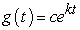where c is the initial population (which is of course 12 thousands in our case) and k is the rate of increase. In this case we only need one point (beside the initial one ) to find the value of “k”. Use the point for t = 1,2,3,4,5 and 6 to find six different model for the exponential function. For each case complete the table for other values (rounded to the nearest thousand) and compare. So for example, for t = 2 the corresponding value of k = 0.24684 (skipping the calculation) and the function model isand the complete table in this case is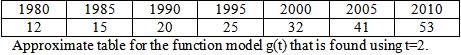Do this for other values of t. Which one is the most accurate? You are encouraged to use the help of a computer and/or calculator to speed up the process.

3.2. The Typical Example and Introduction to the Problem

After the warm-up example and some discussion about it, students are ready for the actual prototype problems. With this example, we will introduce the basic idea of Leslie matrix to the students.

To start with, let us consider certain female population of a specific species of rabbits in the amazon rain forest. Let say that this species of rabbits only has four age groups as follows:

P0 = age 0 = any rabbits that born on the current breeding season.

P1 = age 1 = any 0 age rabbits that is survived to the end of the year and move to the next stage.

P2 = age 2 = any 1 age rabbits that is survived to the end of the year and move to the next stage.

P3 = age 3 = any 2 age rabbits that is survived to the end of the year and move to the next stage.

We will assume that the life cycle of this species of rabbits is ended in these four steps. That is any rabbit that survive in the age group 3 at the end of the year, all (or most of them) will die. The limited number that will survive will not affect the total number and can be ignored.

Now each age group has certain mortality rate and certain reproduction rate. We will now describe this below:

P0 = This group is too young. So this has no reproduction rate.

P1 = Let say that this age group produce an average of 1.2 female rabbits which will survive.

P2 = This is the young generation and thus will have highest rate of reproduction. Let say that this age group produce an average of 1.5 female rabbits which will survive.

P3 = Since this is the oldest age, we may guess that this age group will have lowest rate of reproduction. Let say that this age group produce an average of 0.7 female rabbits which will survive.

We will also make the following assumption about the surviving probability of each age group to the next age group as follows:

50% of P0 population survive from t to t+1

35% of P1 population survive from t to t+1

15% of P2 population survive from t to t+1

0% of P3 population survive from t to t+1 (remember the age group stops at P3 – this is the assumption)

Question_5: Discuss with groups – are these numbers given above make sense? What are some of the factors that can affect the calculation in real life?

In any given time, we will represent the total population as a vector:This represent the population in time “t” of each of the age groups.

We want to find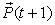fromThis will simply given by the following matrix multiplication (using all the information above):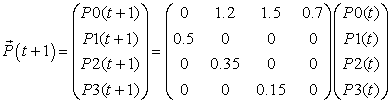Question_6: Group discussionhow the above data that is given fit into the matrix equation above?

This four by four matrix is known as the Leslie matrix. Since we only consider four age groups, we ended up with a four by four Leslie matrix.

Question_7: Let us assume the initial population is given by (in terms of thousand) and the time “t” is measured in years.Use this initial population and the matrix equation to find the population after 1 year, after 2 year.

3.3. Eigenvalues and Eigenvectors of Leslie Matrix: Use of Mathematica

First input the Leslie matrix that we have considered in the previous example.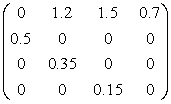In mathematica, we use a list of list to input matrix. So the above matrix is written as:Note that we give the name “L” to this Leslie matrix. The “Eigensystem” command is used to find the set of Eigenvalues and the corresponding Eigenvectors.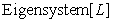Question_8: First show that if A is an n by n matrix and a is a real eigenvalue and V + iU is the corresponding (complex) Eigenvector, where V is the real part and U is the imaginary part, then both V and U are eigenvector of A with eigenvalue a.

Question_9: What is all the positive eigenvalues and the corresponding eigenvectors means in this physical situation? What is all the negative eigenvalues and the corresponding eigenvectors means in this physical situation?

Question_10: Group Discussion - How population modeling with Leslie matrix is different from the population modeling with “polynomial” or “exponential” function?

3.4. Analyze Long Term Behavior of Leslie Matrix

We want to analyze the long term behavior of the Leslie matrix, L. That is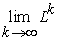We will use the “Table” and “MatrixPower” command of mathematica to do it. To list the first thirty power of L, we will use: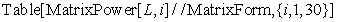Question_11: Group Discussion – Look at the first 30 power of the Leslie matrix. Do you see any limiting behavior? Try to calculate a few more power and analyze more.

We can also calculate the power of matrix applied to a specific initial vector. That is we will start with a given initial population and Leslie matrix and try to find the long term population where the initial population is approaching (if there is any). Now go back to Question_7 and use the same initial condition that is given in that question. We simply use the command:This will give the population after 10 years.

Question_12: Use the command above to find the population after 15 years, after 20 years and after 50 years. What is your conclusion based on this? What will be the long term behavior of the population if the initial population is?

In fact we can do more with mathematica. We can actually use the command: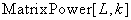This will symbolically calculate the kth power of L. Now we can use the “Limit” command to find the limiting behavior: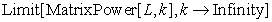Question_13: What answer you got from the previous command?

3.5. Project

Question_14: From the discussion above and also how we generate a Leslie matrix, what is the general shape of a Leslie matrix? Write down a typical example of a 5 by 5 and a 6 by 6 Leslie matrix.

Question_15: Let say some species of age group six, we have the following Leslie matrix: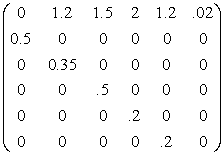Analyze this Leslie matrix. Do all the calculation that is done with our example of 4 by 4 Leslie matrix. Consider different initial population including the following:Question_16: How the entry of a Leslie matrix can be calculated in real life for a particular species? This is not an easy task. What are some of the factors that can affect the finding?

Question_17: What are some of the limitation of modeling population with Leslie type matrix?

Question_18: Leslie matrix is usually uses to study “female” population. Do you see any particular reason for this?

Question_19: Write an example of a situation where you have eight age groups. Write the Leslie matrix and analyze it.

Question_20: How many age group you will have if you want to use Leslie matrix to model human population? Do you think this will be a good model for human population? If not then why not?

3.6. Conclusion

The project at the end can also be used as an assessment tools to measure the student learning outcomes which were mentioned in the beginning of the paper. The modulo can easily be modified, changed and altered to fit the various needs of the students and the instructors.

### Acknowledgement

This project is supported by Us Department of Education Grant DoE -CILES #P031C110158.

### References

  Bailey, T., & Alfonso, M. (2005). Path to persistence: Analysis of research on programs effectiveness at community college. (L. F. education, Ed.) New agenda series, 6(1). Retrieved from http://www.eric.ed.gov/PDFS/ED484239.pdf.In article  Banks, J. (1988). Ethnicity, class, cognitive, and motivational styles in the college classroom. (N. Heights, Ed.) ASHE Reader Series.In article  Engle, J., & Tinto, V. (2008). Moving beyond access: College success for low-income, first generation students. Pell Institue for the Study of Opportunity in Higher Education. Retrieved from http://www.eric.ed.gov/PDFS/ED504448.pdf.In article  McKeachie, W. (1994). Teaching tips: A guidebook for the beginning college teacher (9th ed.). Lexington, MA: Heath.In article  Ormrod, J. E. (2003). Educational Psychology: Developing Learners. Merrill Prentice Hall.In article  Purdue University. (n.d.). A Brief Introduction to Mathematica. Retrieved from http://www.cs.purdue.edu/homes/ayg/CS590C/www/mathematica/math.html.In article  Susan, M., & Linda, N. (1998). Student learning styles and their implications for teaching. Center for research on Learning and Teaching (Occasional Papers)(10).In article  Tiberius, R. (1986). Metaphors underlying the improvement of teaching and learning. British Journal of Educational Technology, 17(2), pp. 144-156.In article CrossRef  wikipedia. (2010). Retrieved from wikipedia.org: http://en.wikipedia.org/wiki/Motivation.In article  Wolfram Mathematica. (2013). Hands-on Start to Mathematica. Retrieved from Wolfram:http://www.wolfram.com/broadcast/screencasts/handsonstart/.In article